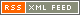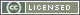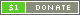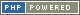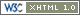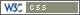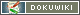# Bitwise Operators

Bitwise Operators perform bitwise operations on the terms specified. When working with integer Numbers, this allows specific bits to be turned on or off. When working with Strings, the ASCII values for the characters are used. When working with Booleans, the effect is like that of using logical operators, except a Boolean value is returned.

Symbol Type Name Description Example
Number
+& Infix AND Bits that are set in both left and right terms are set ` a +& b `
+| Infix OR Bits that are set in either left or right terms are set ` a +| b `
+^ Infix XOR Bits that are set in either left or right terms, but not both, are set ` a +^ b `
+^ Prefix NOT All bits in the term are inverted ` +^ a `
+< Infix Shift left Shift the bits of the left term left by the number of steps specified in the right term ` a +< b `
+> Infix Shift right Shift the bits of the left term right by the number of steps specified in the right term ` a +> b `
String
~& Infix AND Bits that are set in both left and right terms are set ` a ~& b `
~| Infix OR Bits that are set in either left or right terms are set ` a ~| b `
~^ Infix XOR Bits that are set in either left or right terms, but not both, are set ` a ~^ b `
~^ Prefix NOT All bits in the term are inverted ` ~^ a `
~< Infix Shift left Shift the bits of the left term left by the number of steps specified in the right term ` a ~< b `
~> Infix Shift right Shift the bits of the left term right by the number of steps specified in the right term ` a ~> b `
Boolean
?& Infix AND True if left and right terms are true ` a ?& b `
?| Infix OR True if left or right terms are true ` a ?| b `
?^ Infix XOR True if left or right terms, but not both, are true ` a ?^ b `
?^ Prefix NOT Inverts the value of the term ` ?^ a `

Each Operator will evaluate the terms provided as appropriate to the Operator value type - for instance, performing a numerical bitwise OR on two Strings will convert the Strings to Numbers first.